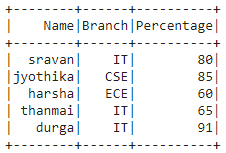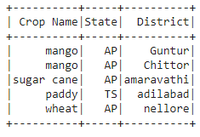# Create PySpark DataFrame from list of tuples

• Last Updated : 30 May, 2021

In this article, we are going to discuss the creation of a Pyspark dataframe from a list of tuples.

To do this, we will use the createDataFrame() method from pyspark. This method creates a dataframe from RDD, list or Pandas Dataframe. Here data will be the list of tuples and columns will be a list of column names.

Syntax:

dataframe = spark.createDataFrame(data, columns)

Example 1:

## Python3

 `# importing module``import` `pyspark`` ` `# importing sparksession from ``# pyspark.sql module``from` `pyspark.sql ``import` `SparkSession`` ` `# creating sparksession and giving``# an app name``spark ``=` `SparkSession.builder.appName(``'sparkdf'``).getOrCreate()`` ` `# list of tuples of college data``data ``=` `[(``"sravan"``, ``"IT"``, ``80``),``        ``(``"jyothika"``, ``"CSE"``, ``85``),``        ``(``"harsha"``, ``"ECE"``, ``60``),``        ``(``"thanmai"``, ``"IT"``, ``65``),``        ``(``"durga"``, ``"IT"``, ``91``)]`` ` `# giving column names of dataframe``columns ``=` `[``"Name"``, ``"Branch"``, ``"Percentage"``]`` ` `# creating a dataframe``dataframe ``=` `spark.createDataFrame(data, columns)`` ` `# show data frame``dataframe.show()`

Output:Example 2:

## Python3

 `# importing module``import` `pyspark`` ` `# importing sparksession from ``# pyspark.sql module``from` `pyspark.sql ``import` `SparkSession`` ` `# creating sparksession and giving ``# an app name``spark ``=` `SparkSession.builder.appName(``'sparkdf'``).getOrCreate()`` ` `# list of tuples of plants data``data ``=` `[(``"mango"``, ``"AP"``, ``"Guntur"``),``        ``(``"mango"``, ``"AP"``, ``"Chittor"``),``        ``(``"sugar cane"``, ``"AP"``, ``"amaravathi"``),``        ``(``"paddy"``, ``"TS"``, ``"adilabad"``),``        ``(``"wheat"``, ``"AP"``, ``"nellore"``)]`` ` `# giving column names of dataframe``columns ``=` `[``"Crop Name"``, ``"State"``, ``"District"``]`` ` `# creating a dataframe``dataframe ``=` `spark.createDataFrame(data, columns)`` ` `# show data frame``dataframe.show()`

Output:Example 3:

Python code to count the records (tuples) in the list

## Python3

 `# importing module``import` `pyspark`` ` `# importing sparksession from``# pyspark.sql module``from` `pyspark.sql ``import` `SparkSession`` ` `# creating sparksession and giving``# an app name``spark ``=` `SparkSession.builder.appName(``'sparkdf'``).getOrCreate()`` ` `#list of tuples of plants data``data ``=` `[(``"mango"``, ``"AP"``, ``"Guntur"``),``        ``(``"mango"``, ``"AP"``, ``"Chittor"``),``        ``(``"sugar cane"``, ``"AP"``, ``"amaravathi"``),``        ``(``"paddy"``, ``"TS"``, ``"adilabad"``),``        ``(``"wheat"``, ``"AP"``, ``"nellore"``)]`` ` `# giving column names of dataframe``columns ``=` `[``"Crop Name"``, ``"State"``, ``"District"``]`` ` `# creating a dataframe ``dataframe ``=` `spark.createDataFrame(data, columns)`` ` `#count records in the list``dataframe.count()`

Output:

`5`

My Personal Notes arrow_drop_up